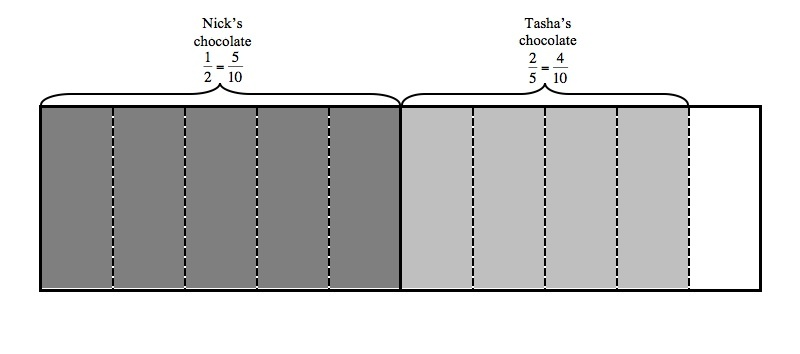# Making S'Mores

Alignments to Content Standards: 5.NF.A.1

## Task

Nick and Tasha are buying supplies for a camping trip. They need to buy chocolate bars to make sâ€™mores, their favorite campfire dessert. Each of them has a different recipe for their perfect sâ€™more. Nick likes to use $\frac{1}{2}$ of a chocolate bar to make a sâ€™more. Tasha will only eat a sâ€™more that is made with exactly $\frac{2}{5}$ of a chocolate bar.

1. What fraction of a chocolate bar will Nick and Tasha use in total if they each eat one sâ€™more?
2. Nick wants to cut one chocolate bar into pieces of equal size so that he and Tasha can make their sâ€™mores. How many pieces should he cut the chocolate bar into so that each person will get the right amount of chocolate to make their perfect sâ€™more?
3. After Nick cuts the chocolate bar into pieces of equal size, how many pieces of the chocolate bar should he get? How many pieces of the chocolate bar should he give to Tasha?

## IM Commentary

The purpose of this instructional task is to motivate a discussion about adding fractions and the meaning of the common denominator. The different parts of the task have students moving back and forth between the abstract representation of the fractions and the meaning of the fractions in the context.

Note that any common multiple of the denominators of the fractions given in this problem could be used in order to find a solution to the addition problem in part (a). It is also important to understand that the common denominator that is used to add the two given fractions corresponds to the number of pieces that Nick will cut the chocolate into in part (b). A classroom discussion of this problem might include the pros and cons for different common denominators when we consider the context of this problem; for example, a smaller denominator will be easier for Nick because he won't have to cut the chocolate bar up into as many pieces.

The Standards for Mathematical Practice focus on the nature of the learning experiences by attending to the thinking processes and habits of mind that students need to develop in order to attain a deep and flexible understanding of mathematics. Certain tasks lend themselves to the demonstration of specific practices by students. The practices that are observable during exploration of a task depend on how instruction unfolds in the classroom. While it is possible that tasks may be connected to several practices, only one practice connection will be discussed in depth. Possible secondary practice connections may be discussed but not in the same degree of detail.Â

This particular task helps illustrate Mathematical Practice Standard 2, Reason abstractly and quantitatively. In the task at hand, fifth graders make sense of fractions and how they are related in a problem situation.Â  They decontextualize the problem and represent it with fractions and symbols. Â As they work through each step, they pause to make sense of the quantities and operations. For instance, students must find a common denominator which really is the number of pieces the chocolate bar is cut into so that both Nick and Tasha can make their sâ€™mores. In part (c) the number of pieces each person receives becomes the numerator of their fractional part of the chocolate bar. Problems such as this one that begin with a context and are represented with mathematical objects or symbols are also examples of modeling with mathematics (MP.4).

## Solution

1. If Nick uses $\frac{1}{2}$ of a chocolate bar and Tasha uses $\frac{2}{5}$ of a chocolate bar, they will use $\frac{1}{2}+\frac{2}{5}$ all together. If we first find a common denominator, we can easily add the fractions. 10 is a common multiple of 2 and 5, so 10 could be used as a common denominator. Since $$\frac{1}{2} = \frac{1\times5}{2\times5} =\frac{5}{10}$$ and $$\frac{2}{5} = \frac{2\times2}{5\times2} = \frac{4}{10}$$ We have that
\begin{align} \frac{1}{2}+\frac{2}{5} &= \frac{5}{10}+\frac{4}{10}\\ &= \frac{9}{10} \end{align}
The picture below illustrates the amount of chocolate they will use:2. In part (a) we used the fact that 10 is a common denominator to write both fractions in terms of tenths. So if Nick cuts the bar into 10 equal sized pieces, each person can use some of those pieces to make their perfect sâ€™more. Note that any common denominator would work, but since all common denominators are multiples of 10, it would be more work for Nick if we used a larger denominator.
3. Since $\frac{1}{2}=\frac{5}{10}$, Nick should take 5 of the ten pieces. Since $\frac{2}{5}=\frac{4}{10}$, Tasha should take 4 of the ten pieces. This is easy to see in the picture shown above.